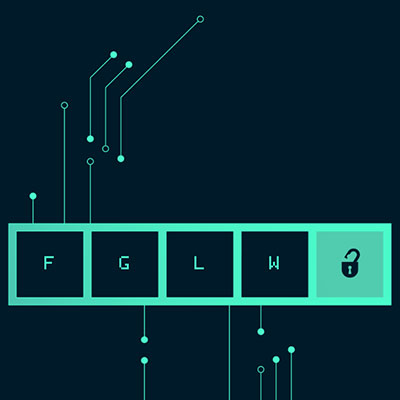# The Guides Section 2 | The Guides Walkthrough | Section 2 Puzzle 7The Guides Walkthrough
Section 2
Puzzle 7

Puzzle 7 is a continuation of Puzzle 6. The lock icon should be unlocked now.
Swipe to the left again to get another clue for this level

This puzzle is simple math. You can substitute any number for the X and complete each step.
To make it ease, we’ll use a 1 for X.

Step 1:
X*2 = Y, so 1*2 = 2

Step 2:
Y+36 = Z, so 2+36 = 38

Step 3:
Z/2 = A, so 38/2 = 19

Step 4:
A-X = B, so 19-1 = 18

Tap the text input field
Key in the number 18
Tap the SUBMIT button